Next: The Quasi-Newton Method. Up: Outline of the Available Previous: The Newton-Raphson Method.

## The Modified Gauss-Newton Method.

From a first guess of the parameters a(1), a sequenceis generated and is intended to converge to a local minimum of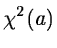. At each iteration, one computes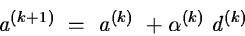where d(k) is a certain descent direction andis a real coefficient which is chosen such that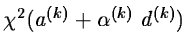is approximately minimum. The direction d(k) is ideally the solution of the Newton equation

H(a(k)d(k) = -g(a(k))

which can also be rewritten

[J(a(k))T J(a(k)) + B(a(k))] d(k) = -J(a(k)r(a(k))  .

Neglecting the second derivatives matrix B(a(k)), we obtain the normal equations'' and the Gauss-Newton direction

J(a(k))T J(a(k)d(k) = -J(a(k)r(a(k))

This so-called Gauss-Newton method is intended for problems where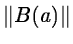is small. If the Jacobian J(a) is singular or near singular or if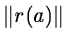is very large (the so-called large residuals problem), the Gauss-Newton equation is not a good approximation of the normal equations and the convergence is not guaranteed.

The algorithm implemented here is a modification of that Gauss-Newton method, that allows convergence even for rank deficient Jacobians or for large residuals. The Gauss-Newton direction is computed in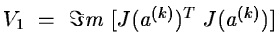, the invariant space corresponding to the non-null eigenvalues. A correction is taken in V2, the orthogonal of V1, according to the second derivatives if the decrease of the objective function at the last iteration is considered too small. The Hessian matrix is estimated using finite differences of the gradient.

This method requires the availability of the derivatives and as the number of gradient evaluations is almost p at each iteration, it is recommended for problems with a small number of parameters, let us say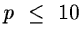Next: The Quasi-Newton Method. Up: Outline of the Available Previous: The Newton-Raphson Method.
Petra Nass
1999-06-09